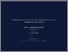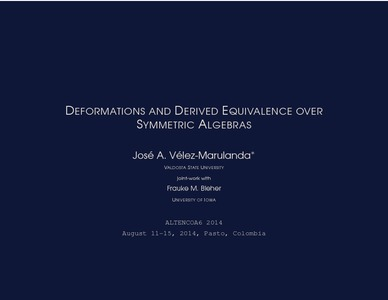# Deformations and Derived Equivalence over Symmetric Algebras

Vélez, José (2014) Deformations and Derived Equivalence over Symmetric Algebras. In: Altencoa6-2014, Agosto 11 al 15 de 2014, Universidad de Nariño - Colombia.Preview
Text
altencoa_p5.pdf

Download (414kB) | Preview

## Resumen

Let k be an algebraically closed field of arbitrary characteristic and let Ʌ be a finite dimensional k-algebra that is also symmetric, i.e. Ʌ and Ʌ*-Homk (Ʌ,k) are isomorphic as Ʌ-bimodules. In the first part of this talk, we discuss the deformation theory of complexes in the derived category D(PCMod(Ʌ)), where PCMod(Ʌ) denotes the abelian category of pseudocompact Ʌ-modules. For the second part of the talk, we assume that T is another symmetric k algebra that is derived equivalent to Ʌ, and we discuss the following statement: if ɅQT is the corresponding split-endomorphism two-sided tilting complex (as introduced by Rickard), then ɅQT preserves the versal deformation rings of complexes in D-(PCMod(Ʌ)), resp. D-(PCMod(T)). This is a joint work with Frauke M. Bleher at the University of Iowa.

Tipo de Elemento: Conferencia o Taller artículo (Speech) Deformations, Derived Equivalence, Symmetric Algebras L Educación > LB Theory and practice of education > LB2300 Higher Education Facultad de Ciencias Exactas y Naturales > Programa de Licenciatura en Matemáticas > Eventos > Álgebra, Teoría de Números, Combinatoria y Aplicaciones Altencoa - 2014 Depto Matemáticas y Estadística 04 Sep 2014 20:27 04 Sep 2014 20:27 http://sired.udenar.edu.co/id/eprint/116Ver Elemento